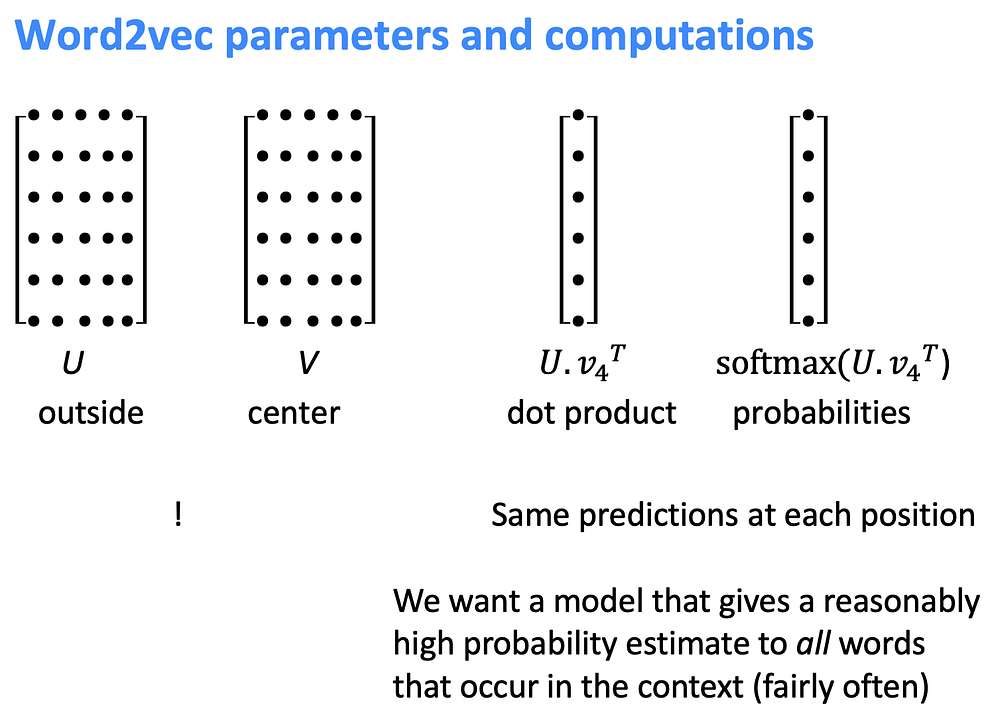# Neural Networks & Word Embeddings

Source: Deep Learning on Medium# Neural Networks & Word Embeddings

All modern NLP techniques use neural networks as a statistical architecture. Word embeddings are mathematical representations of words, sentences and (sometimes) whole documents. Embeddings allow us to compare text, visualize it, and improve the accuracy of newer models. Stanford’s Natural Language Processing with Deep Learning Course is a great course that I’ll follow to get started . For the projects, I’ll complete all 3 coding parts in PyTorch (word2vec and dependency parsing).

# Distributional semantics

Distributional semantics: A word’s meaning is given by the words that frequently appear close-by. In other words “You shall know a word by the company it keeps”.

# Word Embeddings (a.k.a. Word vectors)

We will build a dense vector for each word, chosen so that it is similar to vectors of words that appear in similar contexts [here’s a visual video snippet]. They are a distributed representation. In practice the minimum dimensionality is 50, 300 (on laptop), 1000, 2000, or 4000 are common vector sizes used.

# Word2vec

Word2vec is a framework for learning word vectors. It’s a very simple and scalable way of learning vector representations of words. Ultimately, we want a model that gives a reasonably high probability estimate to all words that occur in the context (fairly often).

## Main Idea/Summary:

1. Iterate through each word of the whole corpus
2. Predict surrounding words using word vectors
3. Update vectors so you can predict well

## More Detailed Steps:

• Every word in a fixed vocabulary is represented by a vector. Start with a random vector for each word.
• Go through each position t in the text, which has a center word `c` and outside context words `o`.
• Use the similarity of the word vectors for `c` and `o` to calculate the probability of `o` given c (or vice versa).
• Keep adjusting the word vectors to maximize this probability.

The question now is how do we calculate the probability of the center word and the context given the center word? [Video Snippet]

We will use two vector representations per word, w. So we will use one vector for a word when it’s the center word and we are predicting other words (v). Then we have a second vector for the word when it’s a context word (u).

NOTE: Generally we may be using Word2vec to get the context of, let’s say, 10 words or so. So it will be a “loose” model where we might get a 5% chance to one of those 10 words being guessed correctly. So while we should not expect to get 97%, the goal is, for example, we hope to capture that the word “withdrawal” is likely to occur with “bank” instead of “football” a non-related word.

# Word2vec the Skip-Gram Model

Here’s an excellent article that covers the skip gram neural network architecture for Word2Vec.

The skip-gram model proposes a simple single-layer architecture based on the inner product between two word vectors. The objective is to predict a word’s context given the word itself.

The intuition of skip-gram is:

1. Treat the target word and a neighboring context word as positive examples.
2. Randomly sample other words in the lexicon to get negative samples.
3. Use logistic regression to train a classifier to distinguish those two cases.
4. Use the regression weights as the embeddings.

[Source: Jurafsky, SLP, Ch 6.8]

Imagine a sentence like the following, with a target word `apricot`, and assume we’re using a window of ±2 context words: